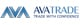USD =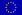EUR =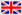GBP =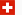CHF =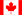CAD =AUD =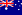NZD =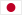JPY =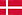DKK =NOK =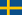SEK =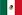MXN =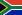ZAR =SGD =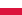PLN =HUF =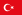TRY =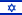ILS =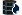OIL =